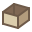Children from resource:basis
 Vocabulary Summarylists.lazy Lazy listsmath.primes Prime numbers test and generationmath.primes.brute-forcemath.primes.erato Eratosthene sievemath.primes.factors Prime factors decomposition

Children from resource:extra
 Vocabulary Summarymath.algebra Various algebra-related wordsmath.continued-fractions Continued fractionsmath.primes.lists Infinite stream of prime numbers through lazy listsmath.text.french Convert integers to French textpath-finding A* path-finding algorithm